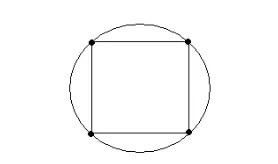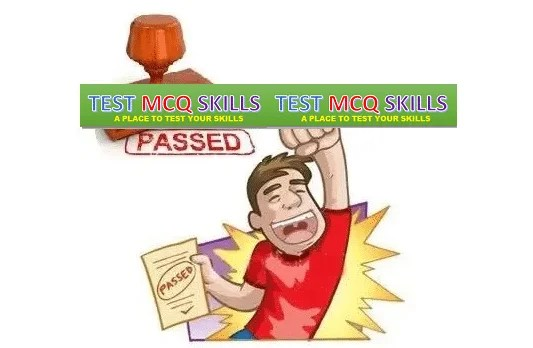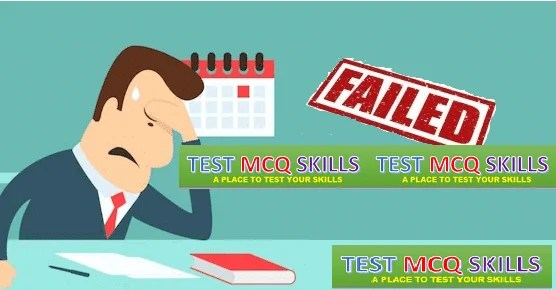Tuesday , October 20 2020
Breaking News

# NTS Quantitative Reasoning Practice Test 4

### NTS Quantitative Reasoning Practice Test # 4

INSTRUCTIONS:

• This Quiz is related to NTS Quantitative Reasoning Practice Test # 4 in this series
• The Quiz based on the Topic, “Quantitative Reasoning
• If you have good understanding about the subject, then you can attempt it
• There will be 10 Multiple Choice Questions (MCQs) in this test
• You have 60 Seconds to answer a single Multiple Choice Question
• Questions will be randomly changed every time you start this test
• You should practice more and more to get high marks
• Passing Criteria is 60 percent for this Quiz
• You can retake this test as many time as you like
• The progress bar at the top of screen will show your progress as well as the time remaining where timed quiz
• After the quiz, You will find Your Test Score and Grade
• If you feel that any Incorrect Answer to a Question, simply Comment us about the Question.

#### The circle in the figure has all its vertices on the circle. The area of the square is 144. What is the area of the circle?Correct! Wrong!

Let x be the side of the square. The area is equal to x2. Hence x2 = 144 , solve for x, x = 12 The diameter d of the circle is equal to the length of the diagonal of the square. Using Pythagora's theorem, we obtain x2 + x2 = d2 Solve for d 144 + 144 = d2 d = 12 √2 The radius r of the circle is equal to d/2 r = 12 √2 / 2 = 6 √2 The area A of the circle is given by A = Pi r2 = 72 Pi

#### The sum of a number x, such that x is greater than 1, and its reciprocal is equal to 10 / 3. Find x. ​

Correct! Wrong!

The reciprocal of x is 1 / x. The sum of x and 1 / x is equal to 10 / 3 is translated mathematically as follows. x + 1 / x = 10 / 3 Multiply all terms in the equation by 3 x and simplify. 3 x2 + 3 = 10x Write the above equation with right hand term equal to 0, factor and solve. 3 x2 - 10 x + 3 = 0 (3x - 1)(x - 3) = 0 Two solutions to the equation x = 1 / 3 and x = 3 We are looking for the solution that is greater than 1. Hence the solution to the problem is x = 3

#### A printer numbered consecutively the pages of a book, beginning with 1 on the first page. In numbering the pages, he had to print a total of 187 digits. Find the number of pages in the book.

Correct! Wrong!

The total number of digits is 187. The total number of single digit page numbers is 9(from 1 to 9). So subtracting this from 187 we get 178. After page number 9 we have 2 digit page numbers. So dividing this by 2 we get 89. So the total number of pages in the book are 89+9=98.

Correct! Wrong!

x4 = 6561

#### In a group of 8 semifinalists, all but 2 will advance to the final round. If in the final round only the top 3 will be awarded medals, then how many groups of medal winners are possible?

Correct! Wrong!

The entire discussion of rounds is a red herring. The question is asking for possible combinations of the final 3, and it is possible for any of the original 8 contestants to have advanced to the final round, thus we need to pick 3 out of 8, and order doesn’t matter. 8*7*6/3*2*1=56.

#### For what values of b and c will the equation x2 + bx = c have the solutions 2 and -3?

Correct! Wrong!

Since x = 2 is a solution of x2 + bx = c, then (2)2 + b(2) = c or 4 + 2b = c Since x = -3 is a solution of x2 + bx = c, then (-3)2 + b(-3) = c or 9 - 3b = c We now have a system of simultaneous equations in b and c to solve. Combining the above equation, we obtain 4 + 2b = 9 - 3b Solve for b 5b = 5 , b = 1 Substitute b by 1 in the equations 4 + 2b = c and solve for c 4 + 2(1) = c or c = 6 Values for b and c b = 1 , c = 6

#### If x = 2 is a solution to the equation (m + 2)x2 = 16, where m is a constant, then what is the second solution of this equation?

Correct! Wrong!

x = 2 is a solution and therefore satisfies the equation. Hence (m + 2)(2)2 = 16 Solve for m 4(m + 2) = 16 m + 2 = 4 m = 2 Substitute m by 2 in the given equation and solve it. (2 + 2)x2 = 16 Substitute m by 2 in the given equation and solve it. 4 x2 = 16 x2 = 4 x = ~+mn~ 2 The second solution is x = - 2

#### Which of the following CANNOT yield an integer when divided by 10? (i) Product of two prime numbers (ii) An integer less than 10 (iii) Sum of three consecutive integers (iv) An odd integer

Correct! Wrong!

This question requires deep thinking in the sense that you have to look for the examples to refute the statements. We will take one by one. If product of two prime numbers when divided by 10 gives us an integer we can remove all answers containing (i). So we take 5 and 2. When multiplied and then divided by 10 we get an integer 1. So (i) is true. Get rid of A. We move to (ii). Lets take 0. This when divided by 10 gives 0, which again is an integer. So (ii) is also true. So get rid of B and C. Now we just need to verify D as it is the obvious choice. Any odd integer when divided by 10 would always leave decimal and never an integer.

#### The marks scored by a student in three subjects are in the ratio of 4: 5: 6. If the candidate scored an overall aggregate of 60% of the sum of the maximum marks and the maximum mark in all three subjects is the same, in how many subjects did he score more than 60%?

Correct! Wrong!

Let the maximum in marks in each of the three subjects be 100. Therefore, the candidate scored an aggregate of 60% of 3 * 100 = 60% of 300 marks = 180 marks. Let the marks scored in the three subjects be 4x, 5x and 6x. Then, 4x + 5x + 6x = 180 ? 15x = 180 or x = 12. Therefore, marks scored by the candidate in the three subjects are 4*12, 5*12 and 6*12 = 48, 60 and 72. Hence, the candidate has score more than 60% in one subject.

#### The product of two positive numbers is p. If each of the numbers is increased by 2, the new product is how much greater than twice the sum of the two original numbers? ​

Correct! Wrong!

Plug in p=10. This can be written as the product of 2 and 5. Their sum is 7. Twice of it is 14. Increasing the original numbers by 2 we get the two numbers as 4 and 7. The product of this is 7*4=28. So the difference between the product and the sum is 28-14=14.This is our target answer. Plug in p=10 back in all the answer choices and look for the answer as 14

GMAT Previous TEST PAPERS-04
Congratulations! You PASSSorry! You FAIL